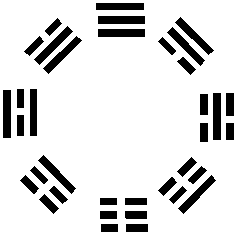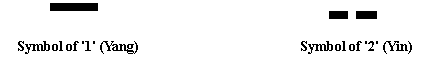## Tuesday, July 29, 2008

### Yin-Yang and Eight Triagrams according to the Book of Changes

Figure 4.4-5 is a symbol that has been used since ancient times in China to symbolize the subdivision of the earth into the '8' stages in the cycle of the moon. This is the written symbol for 'space' in the fourth dimension. The square and the circle are the '2' symbols of the '4' dimensional 'area'. The square is a symbol of subdivision (Yin). The circle has no distinct sides, but seems to have only '1' (Yang) side. The circle is a symbol of unity (Yang). Because space is Yang, the written symbol of space uses only '1' (Yang) symbol of the 'area'. The symbol is in the form of a circle, which symbolizes the Tai-Ji, the Chinese symbol of unity (Yang).Figure 4.4-5

There are '8' symbols that make up the written symbol for space. Each of these symbolizes '1' of the '8' fingers. Each symbol has '3' rows. Each of these rows symbolizes '1' of the bones of the fingers. Half of the segments are composed of '1' (Yang) unified (Yang) segment, and half of the segments are composed of '2' (Yin) subdivided (Yin) segments. (Figure 4.4-6).Found Here: http://5dspace-time.org/Chapter44S.htmlYin-Yang and Eight Triagrams according to the Book of Changes
The Book of Changes is an ancient Chinese book of divination dating from the Zhou dynasty (1100 B.C.-221 B.C.). The Taiji is the symbol of the Tao School, popularly known in the West as the "yin-yang symbol." Taiji evolves into the Eight Triagrams. The Eight Triagrams is a prehistoric diagram thought to disclose the changes of the course of nature. Eight Triagrams multiplied by Eight Triagrams is 64 changes. Each of them has a meaning.The relationship between the site of Chang'an City and divinatory symbols.

The image above shows that the site of Chang'an City had a gentle downward slope from the southeast to the northwest. According to the geographical altitude, it could be divided into 6 levels. After calculations using Book of Changes, each level corresponds to one of the 64 changes. From the best to the worst, the order of each level in the area was ascertained, then the function of each area was decided by the virtue reflected from the meaning of each change.

Founf Here: http://www.pureinsight.org/pi/index.php?news=4731